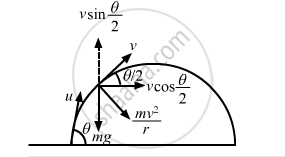Department of Pre-University Education, KarnatakaPUC Karnataka Science Class 11
Advertisement Remove all ads

# What is the Radius of Curvature of the Parabola Traced Out by the Projectile in the Previous Problem at a Point Where the Particle Velocity Makes an Angle θ/2 with the Horizontal? - Physics

Sum

What is the radius of curvature of the parabola traced out by the projectile in the previous problem at a point where the particle velocity makes an angle θ/2 with the horizontal?

Advertisement Remove all ads

#### Solution

Let u be the initial velocity and v be the velocity at the point where it makes an angle $\frac{\theta}{2}$ with the horizontal component. It is given that the horizontal component remains unchanged.
Therefore, we get :

$v \cos \left( \frac{\theta}{2} \right) = u cos\theta$$\Rightarrow v = \frac{u\cos\theta}{\cos\frac{\theta}{2}} . . . \left( i \right)$

$mg\cos\frac{\theta}{2} = \frac{m v^2}{r} . . . \left( ii \right)$

$\Rightarrow r = \frac{v^2}{g\cos\frac{\theta}{2}}$

On substituting the value of v from equation (i), we get :

$r = \frac{u^2 \cos^2 \theta}{g \cos^2 \frac{\theta}{2}}$

Is there an error in this question or solution?
Advertisement Remove all ads

#### APPEARS IN

HC Verma Class 11, 12 Concepts of Physics 1
Chapter 7 Circular Motion
Q 26 | Page 115
Advertisement Remove all ads
Advertisement Remove all ads
Share
Notifications

View all notifications

Forgot password?